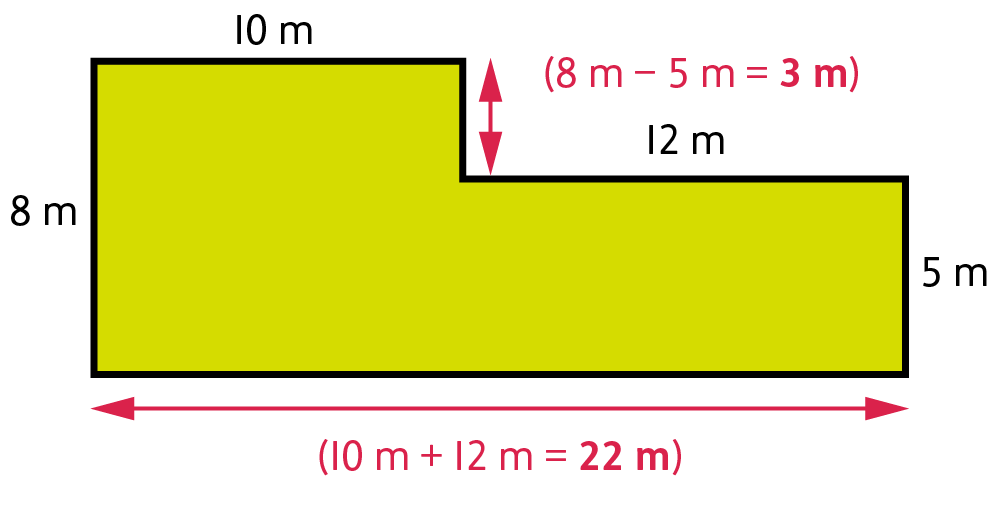We use cookies to enhance your experience on our website. By continuing to use our website, you are agreeing to our use of cookies. You can change your cookie settings at any time. Find out more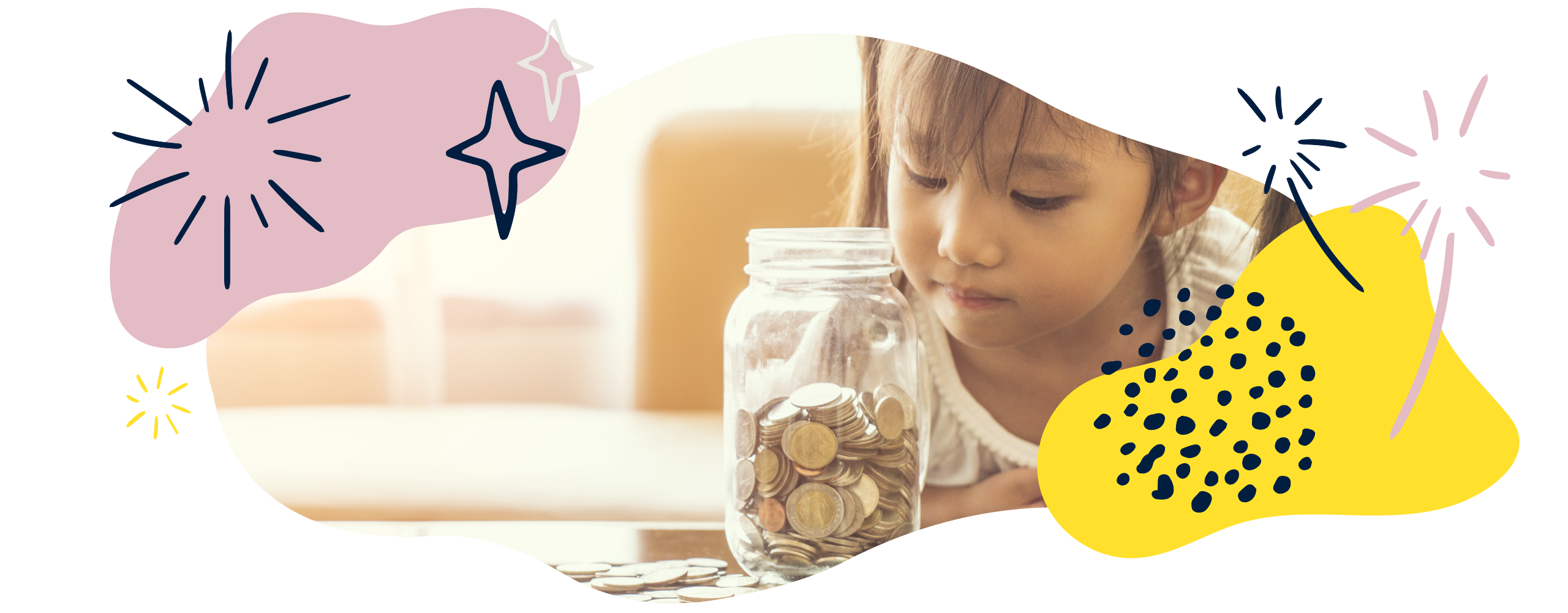# Measurement in Year 5 (Ages 9–10)

In Year 5, your child will convert between metric and imperial units. They will also find the area and perimeter of lots of different shapes, will estimate capacity and volume, and will covert between units of time.

The key words for this section are capacitymassrectilinear, and volume.

## What your child will learn

Take a look at the National Curriculum expectations for measurement in Year 5 (age 9–10):

#### Convert between different units of metric measure

Your child will use their knowledge of place value to convert between standard units. For example:

To convert 1.15kg into grams, you can use your knowledge that 1kg is equivalent to 1000g.

0.15kg is therefore equivalent to 150 grams.

1.15 kg is therefore equivalent to 1150 grams.

Your child should be able to convert between:

• kilometres and metres
• metres and centimetres
• millimetres and centimetres
• kilograms and grams
• litres and millilitres.

#### Use approximate equivalences between metric and common imperial units

Your child will use metric units such as metres, grams, and litres, and imperial units such as inches, pounds, and pints. They will also learn about approximate equivalences between metric and imperial units: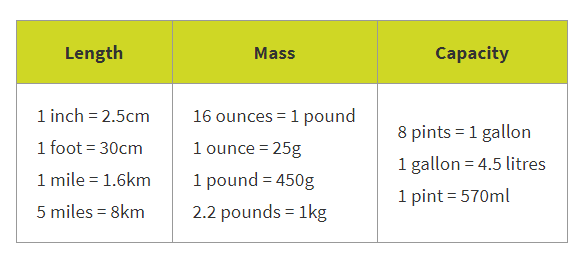#### Measure and calculate the perimeter of composite rectilinear shapes

Your child will measure and calculate the perimeter of rectilinear shapes. These are shapes which have only straight sides and right angles.

Composite shapes are shapes that can be divided into more than one basic shape (for example, a square and a rectangle).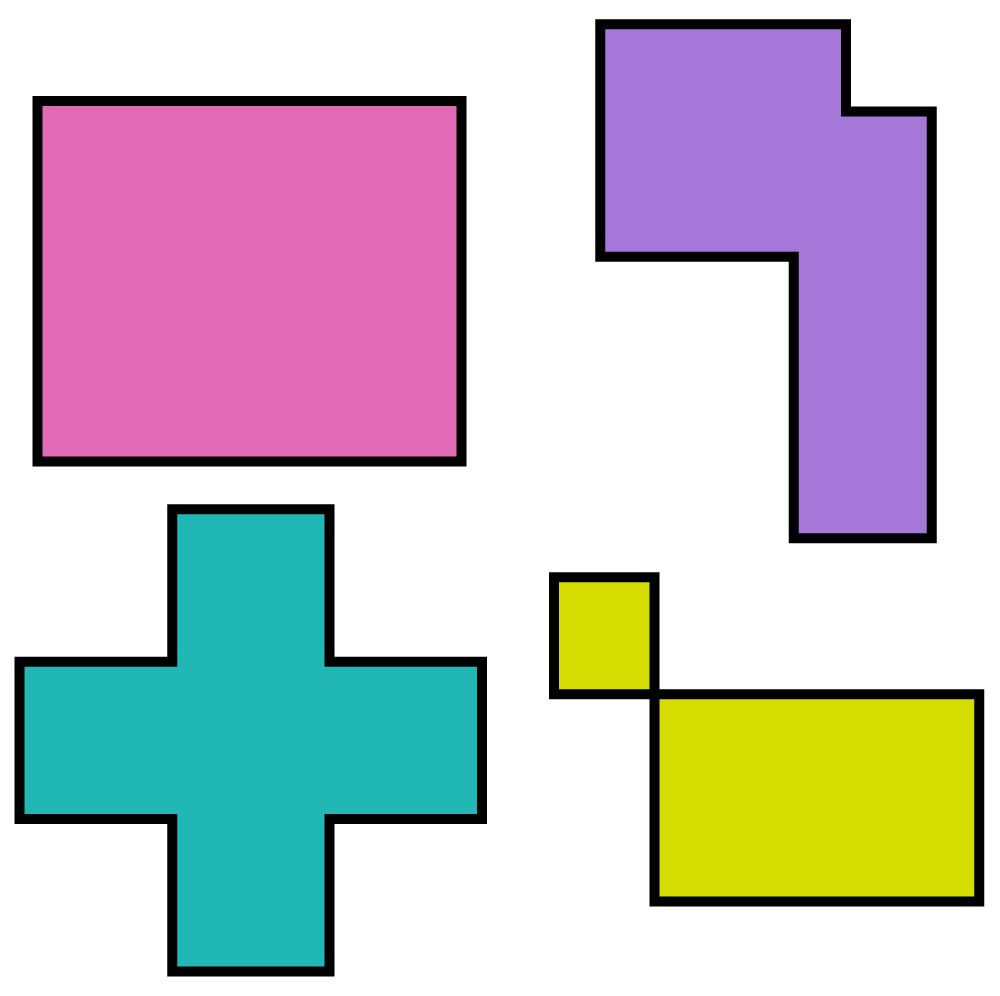Your child will also be able to accurately measure perimeters in millimetres, centimetres, and metres.

#### Calculate the area of rectangles and estimate the area of irregular shapes

Your child will be able to calculate the area of rectangles using standard units, such as square centimetres (cm²) and square metres (m²). They should also be able to calculate area from a scale drawing. For example, they might be given a scaled drawing of a vegetable plot where 1cm is equivalent to 50cm and need to calculate the area.

Your child will use the relationship between perimeter and area to find unknown values. For example:

A square has a perimeter of 36cm. Find the area of the square.

As a square has four equal length sides, we can calculate that each side must be 36cm ÷ 4 = 9cm.

Then, they can calculate the area, which would be 9cm × 9cm which equals 81cm².

Your child will be able to estimate the area of irregular shapes.

#### Estimate volume and capacity

Your child will distinguish between the terms volume and capacity:

• Volume means the amount of space occupied by a 3D shape. It is measured in cubed units like cm³.
• Capacity means the amount a container can hold. Capacity is measured in metric units such as litres or imperial units such as pints.

Your child will estimate the volume and capacity of 3D shapes and containers. They will use objects such as 1 cm³ cubes to work out the volume of 3D shapes.

#### Solve problems involving converting between units of time

Your child will use multiplication and division to convert between units of time. For example:

Convert 180 minutes into hours.

180 ÷ 60 = 3 hours

#### Use all four operations to solve measure problems using decimal notation

Your child will use addition, subtraction, multiplication, and division to solve problems involving:

• length
• mass
• volume
• capacity
• time
• money.

These problems will include:

• Conversions – for example, ‘Convert 24 days into weeks, expressing the answer as weeks and days.’
• Scaling – for example, ‘If an item costs £28.40 and there is a 50% discount, what is the new price?’
• Decimals – for example, ‘If one plank of wood measures 1·6 m and four planks of wood are needed, what is the total length of wood needed?’

## How to help at home

There are lots of everyday ways you can help your child to understand measurement. Here are just a few ideas.

### 1. Understand the difference between capacity and volume

The difference between volume and capacity is quite subtle, and can be difficult to understand:

• Volume means the amount of space occupied by a 3D shape. It is measured in cubed units like cm³.
• Capacity means the amount a container can hold. Capacity is measured in metric units such as litres or imperial units such as pints.

You could explore capacity with your child using a 1l jug. Gather together some cups and containers. Can your child estimate the capacity of each container? Check how close their estimate was using the 1l jug.

### 2. Convert between units of measurement

• metric units such as kilograms, litres and metres
• imperial units such as inches, pounds, and pints.

Your child may be less familiar with imperial units. Ask them if they know of any items that use imperial measurements – for example, milk is usually sold in pints and the weight of people is often measured in stones and pounds. Point out these examples when you spot them in real life.

On your next trip to the supermarket, ask your child to do any weighing. Can they tell you the weight of some fruit or vegetables in grams and kilograms? Can they convert between metric measurements (like grams) and imperial measurements (like ounces)?

A table like this is useful for getting to grips with approximate equivalences between metric and imperial units:### 3. Practise managing money

Budgeting with precise amounts of money can make for great calculating practice.

Try involving your child in some budgeting activities – for example, budgeting for a family day out. Tell them how much they have to spend, and ask them to work out the cost of different options.

You could also get your child used to budgeting by giving them a small amount of pocket money each week. If there is something your child particularly wants to buy, ask them to think about how much money they will need to save every week.

### Activity: My money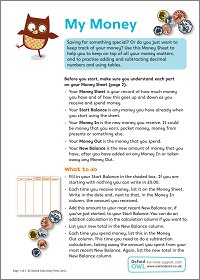Keep on top of all your money matters.

### 4. Understand perimeter and area

Perimeter means the distance around the outside of a shape. Area is the size a surface takes up. Your child will learn to find the perimeter and area of rectilinear shapes, which are shapes with straight sides and right angles.

When finding the area of composite rectilinear shapes (shapes that can be divided into more than one rectangle), encourage your child to divide the shape into smaller squares and rectangles. Here is an example of finding the area and perimeter of a composite rectilinear shape:

#### Area

To find the area of the total shape, your child will need to separate the shape into two rectangles: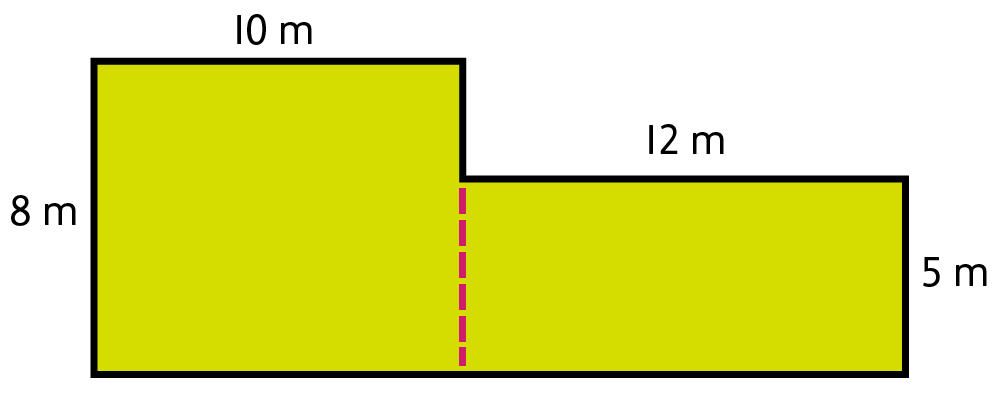The total area of the shape can be found by adding the area of the two rectangles.

10m × 8m = 80m²

12m × 5m = 60m²

80m² + 60m² = 140m²

#### Perimeter

To find the perimeter, your child will first need to find the lengths of the missing sides: